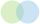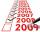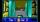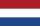Lines

How many lines can be draw with 8 points, if three points lie on one line and the other any three points do not lie on the same line?

Result

n =  26

Solution:Leave us a comment of example and its solution (i.e. if it is still somewhat unclear...):Be the first to comment!To solve this verbal math problem are needed these knowledge from mathematics:

Would you like to compute count of combinations? See also our variations calculator.

Next similar examples:

1. Points in planeThe plane is given 12 points, 5 of which is located on a straight line. How many different lines could by draw from this points?
2. LinesIn how many points will intersect 14 different lines, where no two are parallel?
3. LineIt is true that the lines that do not intersect are parallel?
4. ChordsHow many 4-tones chords (chord = at the same time sounding different tones) is possible to play within 7 tones?
5. ExaminationThe class is 21 students. How many ways can choose two to examination?
6. BlocksThere are 9 interactive basic building blocks of an organization. How many two-blocks combinations are there?
7. Fish tankA fish tank at a pet store has 8 zebra fish. In how many different ways can George choose 2 zebra fish to buy?
8. Volleyball8 girls wants to play volleyball against boys. On the field at one time can be six players per team. How many initial teams of this girls may trainer to choose?
9. TrinityHow many different triads can be selected from the group 43 students?
10. TeamsHow many ways can divide 16 players into two teams of 8 member?
11. ConfectioneryThe village markets have 5 kinds of sweets, one weighs 31 grams. How many different ways a customer can buy 1.519 kg sweets.
12. SubsetsHow many 19 element's subsets can be made from the 26 element set?Determine the number of integers from 1 to 106 with ending four digits 2006.We want to prove the sentence: If the natural number n is divisible by six, then n is divisible by three. From what assumption we started?Four pupils is going via dark hall. Start at one side and have to get to the other side for 17 minutes. They have only one lamp for the trip. The corridor is narrow, they can therefore go up two pupils at a time and go at the slower speed. Each pupil went.Calculate: ?From the colors - red, blue, green, black and white, create all possible tricolors.